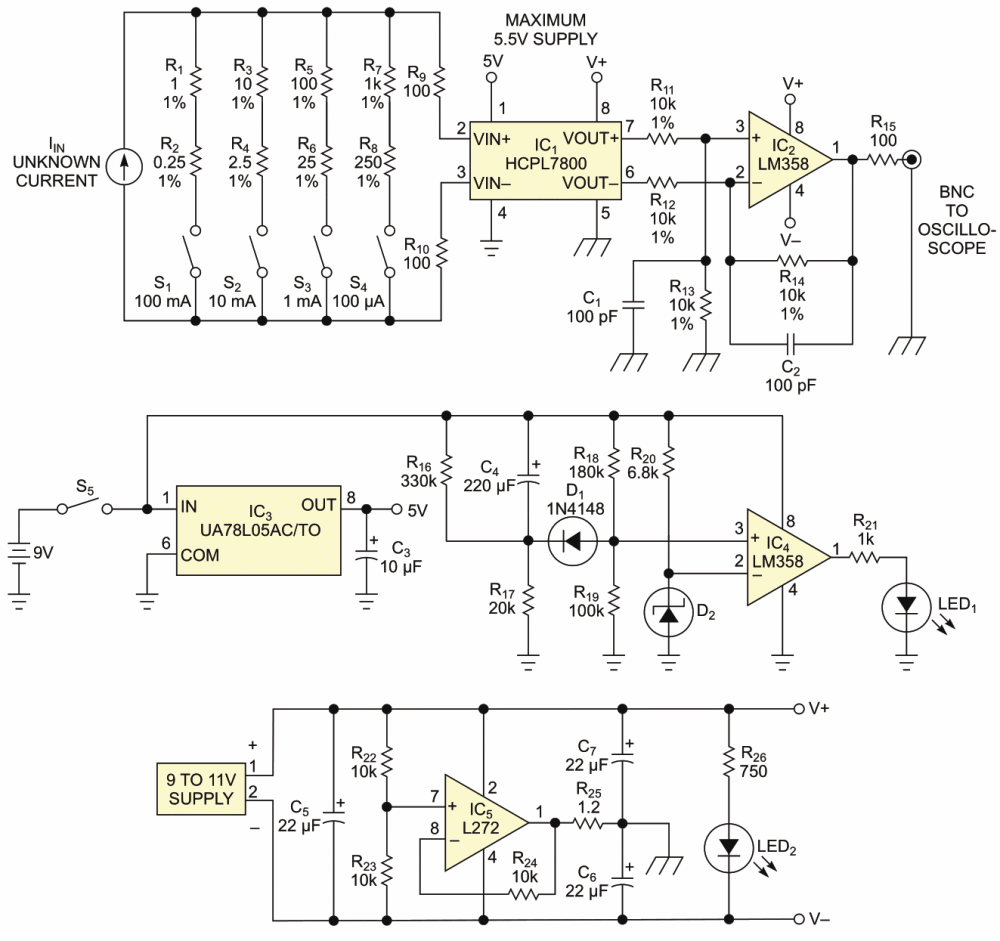# Circuit lets you isolate and measure current

## STMicroelectronics L272 LM358

You often need to measure current during circuit design and debugging. You can perform that task by breaking a path, inserting a shunt resistor, measuring its voltage, and converting the voltage to current. Unfortunately, that approach is sometimes impractical with an oscilloscope because one side of an oscilloscope probe connects to ground. Thus, you need to isolate the oscilloscope from the circuit under test.Figure 1. This circuit produces a voltage proportional to current and isolates the oscilloscope from the measurement point.

The circuit in the Figure 1 produces a voltage proportional to current and isolates the oscilloscope from the measurement point. The circuit uses IC1, an HCPL-7800 isolation amplifier, which adds input-to-output isolation of as much as 890 V. It also amplifies its input voltage by eight. The Table 1 shows the overall gain for each input range. The circuit’s bandwidth is typically 100 kHz.

Table 1. Gain for each input range
 Switch Gain Maximum input current S1 1 V/ 100 mA 160 mA S2 1 V/ 10 mA 16 mA S3 1 V/ 1 mA 1.6 mA S4 1 V/ 100 μA 160 μA

A set of switches lets you select a range of current to measure by inserting resistors into the circuit. Use resistors with 1% or less tolerance to minimize errors. For example, when you close S4, you select the 100-μA range. The unknown current passes through serial resistors R7 and R8, which have values of 1 and 0.25 kΩ, respectively. Thus, the voltage at IC1’s inputs is IIN × 1.25 kΩ; if the input current is 100 μA, the voltage at IC1 is 125 mV. The circuit has a gain of eight, yielding 125 mV × 8, or 1 V. The LM358 acts as a unity-gain differential amplifier. For best linearity, the input voltage at IC1 should not exceed ±200 mV.

The HCPL-7800 has a 3% tolerance. When you are using resistors with 1% tolerance, the 3% tolerance dominates the overall uncertainty of the circuit. The circuit uses two independent voltage supplies. A 9-V battery supplies the input part of IC1. A stabilized 9-V-to-11-V wall-wart power supply powers the output side of IC1 with IC2, an LM358 successive amplifier.

When battery switch S5 closes and the voltage of the battery is sufficient for the circuit, LED1 illuminates for approximately 3 s. The duration of this illumination minimizes drain on the battery. LED2 is on when the 9-V-to-11-V power supply is operating. IC5, an L272, provides an additional ground potential halfway between the supply voltage. With this split supply, you can measure both positive and negative currents.

EDN